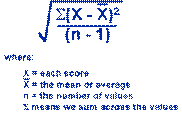Research l Reviews l Theories l Mental Health l QuizNursing Research Quiz - 1
Biostatistics
 1 2 3 4 5 6 7 8 9 10 11 12 13 14 15 16 17 18 19 20 21 22 23 24 25 26 27 28 29 301. Random sampling or probability sampling includes all the following techniques, except:

A. Simple random sampling

B. Stratified random Sampling

C. Cluster sampling

D. Purposive Sampling

2. Gender, age-class, religion, type of disease, and blood group are measured on:

A. Nominal scale of measurement

B. Ordinal scale of measurement

C. Interval scale of measurement

D. Ratio scale of measurement

3. Which scale of measurement has an absolute zero?

A. Nominal

B. Ordinal

C. Interval

D. Ratio

4. The variable which is influenced by the intervention of the researcher is called:

A. Independent

B. Dependent

C. Discrete

D. Extraneous

5. The statistical approach which helps the investigator to decide whether the outcome of the study is a result of factors planned within design of the study or determined by chance is called:

A. Descriptive statistics

B. Inferential statistics

C. Normal distribution

D. Standard deviation

6. Which of the following methods is a form of graphical presentation of data?

A. Line Diagram

B. Pie diagram

C. Bar diagram

D. Histogram

7. All the following are measures of central tendency, except:

A. Mean

B. Median

C. Mode

D. Variance

8. Which measure of central tendency is Influenced by extreme scores and skewed distributions?

B. Median

C. Mode

D. Range

9. A measure of central tendency which is calculated by numbers arranging in numerical order is:

A. Standard deviation

B. Range

D. Mode

10. The proportion of observations fall above the median is:

A. 68%

B. 50%

C. 75%

D. 95%

11. The indices used to measure variation or dispersion among scores are all, except:

A. Range

B. Variance

C. Standard deviation

D. Mean

12. A measure of dispersion of a set of observations in which it is calculated by the difference between the highest and lowest values produced is called:

A. Standard deviation

C. Range

D. Mode

13. A statistic which describes the interval of scores bounded by the 25th and 75th percentile ranks is:

A. Inter quartile range

B. Confidence Interval

C. Standard deviation

14. The Median value is the:

A. 25th percentile

B. 50th percentile

C. 75th percentile

D. 95th percentile

15. Large standard deviations suggest that:

A. scores are probably widely scattered.

B. there is very little deference among scores.

C. mean, median and mode are the same

D. the scores not normally distributed.

16. The formula given below is computational formula for:A. Variance

C. Standard deviation

D. t-statistic

17. The squire of the standard deviation is the:

A. Variance.

B. Standard error

D. Variance

18. Which is NOT a characteristic of normal distribution?

B. Bell-shaped

C. Mean = median = mode

D. Negative skewness

19. Skewness is a measure:

A. of the asymmetry of the probability distribution

B. decides the distribution may have high or low variance

C. of central tendency

D. None of the above

20. The formula given below is used to calculate:O= Observed frequency E= Expected frequency

A. t-test statistic

B. chi-squire statistic

C. correlation coefficient

D. Standard deviation